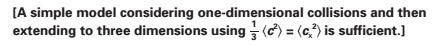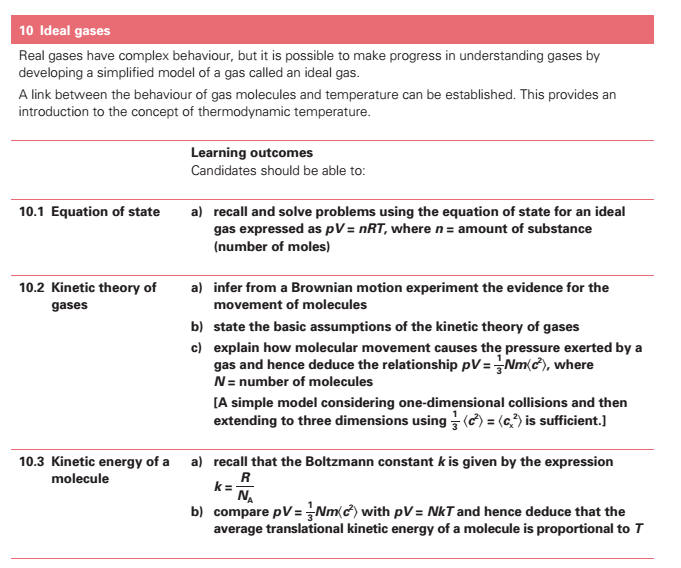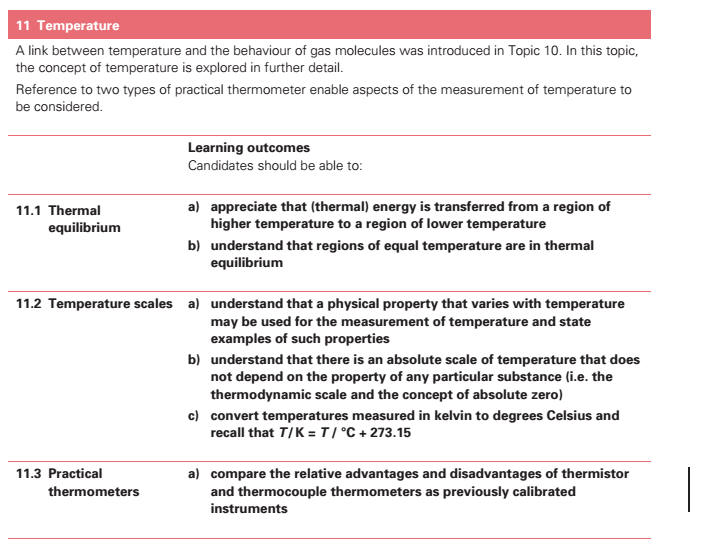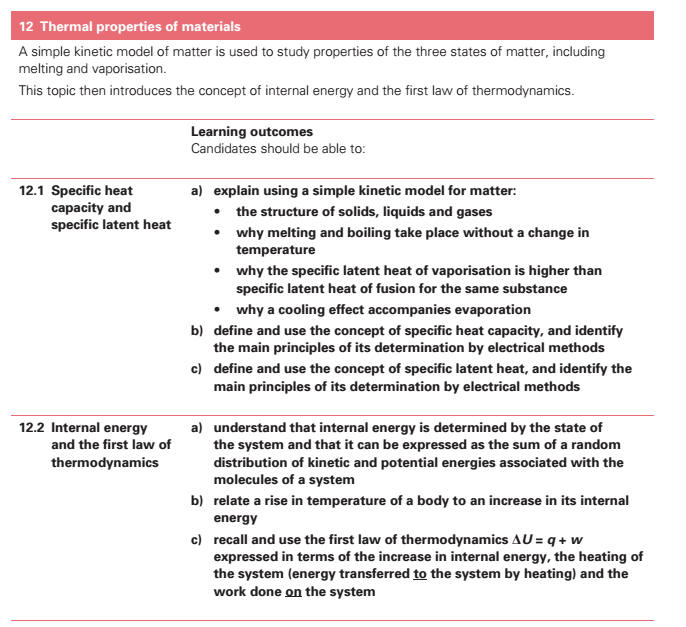11. Ideal Gases[] (b) relate the difference in the structures and densities of solids, liquids and gases to simple ideas of the spacing, ordering and motion of molecules. (c) describe a simple kinetic model for solids, liquids and gases. (d) describe an experiment which demonstrates Brownian motion[] and appreciate the evidence for the movement of molecules provided by such an experiment. (a) recall and solve problems using the equation of state for an ideal gas expressed as pV = nRT. (n = number of moles) (b) infer from a Brownian motion[] experiment the evidence for the movement of molecules. (c) state the basic assumptions of the kinetic theory[] of gases. (d) explain how molecular movement causes the pressure[] exerted by a gas and hence deduce the relationship, pV = (1/3)Nm2 >  (N = number of molecules)(e) compare pV = (1/3)Nm2 > with pV = NkT and hence deduce that the average translational kinetic energy[] of a molecule is proportional to T. 12. Temperature[] (a) show an appreciation that thermal energy is transferred from a region of higher temperature to a region of lower temperature. (b) show an understanding that regions of equal temperature[] are in thermal equilibrium[]. (c) show an understanding that a physical property which varies with temperature[] may be used for the measurement of temperature[] and state examples of such properties. * (d) compare the relative advantages and disadvantages of resistance[] and thermocouple[] thermometers as previously calibrated instruments. (e) show an understanding that there is an absolute scale of temperature[] which does not depend on the property of any particular substance (i.e. the thermodynamic scale and the concept of absolute zero). (f) convert temperatures measured in kelvin to degrees Celsius: T / K = T / °C + 273.15. 13. Thermal Properties of Materials (a) explain using a simple kinetic model for matter why (i) melting and boiling take place without a change in temperature, (ii) the specific latent heat of vaporisation is higher than specific latent heat[] of fusion[] for the same substance, (iii) a cooling effect accompanies evaporation[]. (b) define and use the concept of specific heat capacity[], and identify the main principles of its determination by electrical methods. (c) define and use the concept of specific latent heat, and identify the main principles of its determination by electrical methods. (d) relate a rise in temperature[] of a body to an increase in its internal energy. (e) show an understanding that internal energy[] is determined by the state of the system and that it can be expressed as the sum of a random distribution of kinetic and potential energies associated with the molecules of a system. (f) recall and use the first law of thermodynamics expressed in terms of the change in internal energy, the heating of the system and the work done[] on the system.